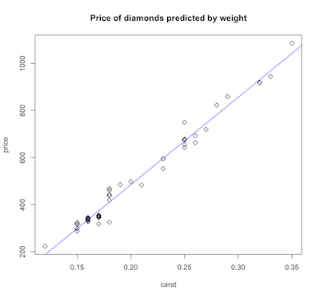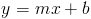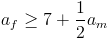## Tuesday, August 31, 2010

### Probability processor

MIT's Technology Review reports on A New Kind of Microchip, a probability-based processor designed to speed up statistical computations.

The chip works with electrical signals that represent probabilities, instead of 1s and 0s using building blocks known as Bayesian NAND gates. “Whereas a conventional NAND gate outputs a "1" if neither of its inputs match, the output of a Bayesian NAND gate represents the odds that the two input probabilities match. This makes it possible to perform calculations that use probabilities as their input and output.”

“This is not digital computing in the traditional sense,” says Ben Vigoda, founder of Lyric Semiconductor. “We are looking at processing where the values can be between a zero and a one.” (from Wired article Probabilistic Chip Promises Better Flash Memory, Spam Filtering) Vigoda's Analog Logic: Continuous-Time Analog Circuits for Statistical Signal Processing probably spells it all out, if you've got the fortitude to read it. For us light-weights, there's a video Lyric Semiconductor explains its probability chip. It's super-cool that he mentions genomics as a potential application.

Computing has been steadily moving towards more specialized coprocessors, for example the vector capabilities of graphics chips (GPU's). Wouldn't it be neat to have a stats coprocessor alongside your general purpose CPU? (Or inside it like an FPU?) How about a cell processor configuration where you'd get an assortment of CPU cores, graphic/vector GPU cores and probability processor cores?

## Saturday, August 21, 2010

### Using R for Introductory Statistics, Chapter 3.4

...a continuing journey through Using R for Introductory Statistics, by John Verzani.

#### Simple linear regression

Linear regression is a kooky term for fitting a line to some data. This odd bit of terminology can be blamed on Sir Francis Galton, a prolific victorian scientist and traveler who saw it as related to his concept of regression toward the mean. Calling it a linear model is a little more straight-forward, and linear modeling through the lm function is bread-and-butter to R.

For example, let's look at the data set diamonds to see if there's a linear relationship between weight and cost of diamonds.```f = price ~ carat
plot(f, data=diamond, pch=5,
main="Price of diamonds predicted by weight")
res = lm(f, data=diamond)
abline(res, col='blue')
```

We start by creating the formula f using the strange looking tilde operator. That tells the R interpreter that we're defining a symbolic formula, rather than an expression to be evaluated immediately. So, our definition of formula f says, "price is a function of carat". In the plot statement, the formula is evaluated in the context given by data=diamond, so that the variables in our formula have values. That gives us the scatter plot. Now let's fit a line using lm, context again given by data=diamond, and render the resulting object as a line using abline. Looks spiffy, but what just happened?

The equation of a line that we learned in high school is:Minimizing squared error over our sample gives us estimates of the slope and intercept. The book presents this without derivation, which is a shame.

Maybe later, I'll get brave an try to insert a derivation here.

#### Examples

There's a popular linear model that applies to dating, which goes like this: It's OK for a man to date a younger woman if her age is at least half the man's age plus seven. In other words, this:Apparently, I should be dating a 27 year old. Let me go ask my wife if that's OK. In the meantime, let's see how our rule compares to results of a survey asking the proper cutoff for dating for various ages.

```plot(jitter(too.young\$Male), jitter(too.young\$Female),
main="Appropriate ages for dating",
xlab="Male age", ylab="Female age")
abline(7,1/2, col='red')
res <- lm(Female ~ Male, data=too.young)
abline(res, col='blue', lty=2)
legend(15,45, legend=c("half plus 7 rule",
"Estimated from survey data"),
col=c('red', 'blue'), lty=c(1,2))
```

That's a nice correspondence. On second thought, this is statistical proof that my daughter is not allowed to leave the house 'til she's 30.

Somehow related to that is the data set Animals, comparing weights of body and brain for several animals. The basic scatterplot not revealing much, we put the data on a log scale and find that it looks much better. As near as I can tell, the I or AsIs function does something like the opposite of the tilde operator. It tells the interpreter to go ahead and evaluate the enclosed expression. The general gist is to transform our data to log scale then apply linear modeling.

```f = I(log(brain)) ~ I(log(body))
plot(f, data=Animals,
main="Animals: brains vs. bodies",
xlab="log body weight", ylab="log brain weight")
res = lm(f, data=Animals)
abline(res, col='brown')
```Now the problem is, the line doesn't seem to fit very well. Those three outliers on the right edge have high body weights but less than expected going on upstairs. That seems to unduly influence the linear model away from the main trend. R contains some alternative algorithms for fitting a line to data. The function lqs is more resistant to outliers, like the large but pea-brained creatures in this example.

```res.lqs = lqs(f, data=Animals)
abline(res.lqs, col='green', lty=2)
```That's better. Finally, you might use identify to solve the mystery of the knuckleheaded beasts.

```with(Animals, identify(log(body), log(brain), n=3, labels=rownames(Animals)))
```

Problem 3.31 is about replicate measurements, which might be a good idea where measurement error, noisy data, or other random variation is present. We follow the by now familiar procedure of defining our formula, doing a scatterplot, building our linear model, and finally plotting it over the scatterplot.We are then asked to look at the variance of measurements at each particular voltage. To do that, we'll first split our data.frame up by voltage. The result is a list of vectors, one per voltage level.

```breakdown.by.voltage = split(breakdown\$time, breakdown\$voltage)
str(breakdown.by.voltage)
List of 7
\$ 26: num [1:3] 5.8 1580 2323
\$ 28: num [1:5] 69 108 110 426 1067
\$ 30: num [1:11] 7.7 17 20 21 22 43 47 139 144 175 ...
\$ 32: num [1:15] 0.27 0.4 0.69 0.79 2.75 3.9 9.8 14 16 27 ...
\$ 34: num [1:19] 0.19 0.78 0.96 1.31 2.78 3.16 4.15 4.67 4.85 6.5 ...
\$ 36: num [1:15] 0.35 0.59 0.96 0.99 1.69 1.97 2.07 2.58 2.71 2.9 ...
\$ 38: num [1:7] 0.09 0.39 0.47 0.73 1.13 1.4 2.38
```

Next, let's compute the variance for each component of the above list and build a data.frame out of it.

```var.by.voltage = data.frame(voltage=names(breakdown.by.voltage),
variance=sapply(breakdown.by.voltage,
FUN=var))
```

This split-apply-combine pattern looks familiar. It's basically a SQL group by in R. It's also the basis for Hadley Wickham's plyr library. Plyr's ddply function takes breakdown, a data.frame, and splits it on values of the voltage column. For each part, it computes the variance in the time column, then assembles the results back into a data.frame.

```ddply(breakdown, .(voltage), .fun=function(df) {var(df\$time)})
```

While that's not directly related to linear modeling, this kind of exploratory data manipulation is what R is made for.

More fun

Previous episode of Using R for Introductory Statistics

## Wednesday, August 11, 2010

### Using R for Introductory Statistics 3.3

...continuing our way though John Verzani's Using R for introductory statistics. Previous installments: chapt1&2, chapt3.1, chapt3.2

#### Relationships in numeric data

If two data series have a natural pairing (x1,y1),...,(xn,yn), then we can ask, “What (if any) is the relationship between the two variables?” Scatterplots and correlation are first-line ways of assessing a bivariate data set.

#### Pearson's correlation

The Pearson's correlation coefficient is calculated by dividing the covariance of the two variables by the product of their standard deviations. It ranges from 1 for perfectly correlated variables to -1 for perfectly anticorrelated variables. 0 means uncorrelated. Independent variables have a correlation coefficient close to 0, but the converse is not true because the correlation coefficient detects only linear dependencies between two variables. [see wikipedia entry on correlation]Question 3.19 concerns a sampling of 1000 New York Marathon runners. We're asked whether we expect a correlation between age and finishing time.

```attach(nym.2002)
cor(age, time)
 0.1898672
cor(age, time, method="spearman")
0.1119944
```

We discover a low correlation - good news for us wheezing old geezers. A scatterplot might show something. And we have the gender of each runner, so let's use that, too.

First, let's set ourselves up for a two panel plot.

```par(mfrow=c(2,1))
par(mar=c(2,4,4,2)+0.1)
```

Next let's set up colors - pink for ladies, blue for guys - and throw in some transparency because a lot of data points are on top of each other.

```blue = rgb(0,0,255,64, maxColorValue=255)
pink = rgb(255,192,203,128, maxColorValue=255)

color <- rep(blue, length(gender))
color[gender=='Female'] <- pink
```

In the first panel, draw the scatter plot.

```plot(time, age, col=color, pch=19, main="NY Marathon", ylim=c(18,80), xlab="")
```

And in the second panel, break it down by gender. It's a well kept secret that outcol and outpch can be used to set the color and shape of the outliers in a boxplot.

```par(mar=c(5,4,1,2)+0.1)
boxplot(time ~ gender, horizontal=T, col=c(pink, blue), outpch=19, outcol=c(pink, blue), xlab="finishing time (minutes)")
```

Now return our settings to normal for good measure.

```par(mar=c(5,4,4,2)+0.1)
par(mfrow=c(1,1))
```Sure enough, there doesn't seem to be much correlation between age and finishing time. Gender has an effect, although I'm sure the elite female runners would have little trouble dusting my slow booty off the trail.

It looks like we have fewer data points for women. Let's check that. We can use table to count the number of times each level of a factor occurs, or in other words, count the number of males and females.

```table(gender)
gender
Female   Male
292    708
```

I'm still a little skeptical of our previous result - the low correlation between age and finishing time. Let's look at the data binned by decade.

```bins <- cut(age, include.lowest=T, breaks=c(20,30,40,50,60,70,100), right=F, labels=c('20s','30s','40s','50s','60s','70+'))
boxplot(time ~ bins, col=colorRampPalette(c('green','yellow','brown'))(6), ylim=c(570,140))
```It looks like you're not washed up as a runner until your 50's. Things go down hill from there, but, it doesn't look very linear, so we shouldn't be too surprised about our low r.

Coarser bins, old and young using 50 as our cut-off, reveal that there's really no correlation in the younger group. In the older group, we're starting to see some correlation. I suppose you could play with the numbers to find an optimum cut-off that maximized the difference in correlation. Not sure what the point of that would be.

```y <- nym.2002[age<50,]
cor(y\$age,y\$time)
 -0.01148919
cor(y\$age,y\$time, method='spearman')
 -0.01512368
```
```o <- nym.2002[age>=50,]
cor(o\$age, o\$time)
 0.3813543
cor(o\$age, o\$time, method='spearman')
 0.1980635
```

I ran a marathon once in my life. I think I was 30 and my time was a pokey 270 or so. My knees hurt for days afterwards, so I'm not sure I'd try it again. I do want to do a half, though. Gotta get back in shape for that...